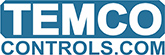Here is the example of custom clock in which you can calculate seconds, minutes etc

10 REM “CLOCK”
20 IF SYS_TIME <> TIME THEN GOTO 30 ELSE GOTO 80
30 SECONDS = SECONDS + 1
40 IF SECONDS >= 59 THEN MINUTES = MINUTES + 1 , SECONDS = 0
50 IF MINUTES = 59 THEN HOURS = HOURS + 1 , MINUTES = 0
60 IF HOURS = 23 THEN DAYS = DAYS + 1 , HOURS = 0
70 IF DAYS > 30 THEN DAYS = 0
80 SYS_TIME = TIME

Thanks for the tips here waheedj, its appreciated. This would be useful for months with 30 days and you could work up some logic to do exceptions on the other months. Then there’s daylight savings and leap years to deal with… !

You can also have a look at the DOM, DOW, DOY statements which returns the day of week, month and year. Then there’s the TIME command which you used, it returns a real value with the hours in the first two digits, minutes in the next two and seconds after the decimal point: 14:30:30 returns a value of 1430.30

Time command returns time in seconds, so 14:30:30 would return 52230.
Looking at the program above, minutes are 59 seconds long, hours are 59 minutes long and days are 23 hours long.
If I need to check controller’s time from external device I read controller’s registers.
Example: Reg200 - seconds, Reg201 - minutes, Reg202 - hours
If I need hours, minutes and seconds calculated within a program I use this:

10 HOURS = INT (Time / 3600)
20 CON1 = HOURS * 3600
30 CON2 = TIME - CON1
40 MINUTES = INT (CON2 / 60)
50 CON3 = MINUTES * 60
60 SECONDS = TIME - CON1 - CON3

CONs are value holding registers for calculations. I use CONs so I don’t waste VARs.
For days DOM, DOW, DOY commands.

1 Like

Yes Maurice lot of things can be done on this and will be very useful.